## Regression

• Supervised learning refers to algorithms that learn $$x \rightarrow y$$ aka input to output mappings.
• It learns the correct label $$y$$ for given input $$x$$.
• It’s by seeing correct pairs of $$x$$ and $$y$$, supervised learning models learns to take just the input alone and give a reasonable output $$y$$.
• Example use cases below: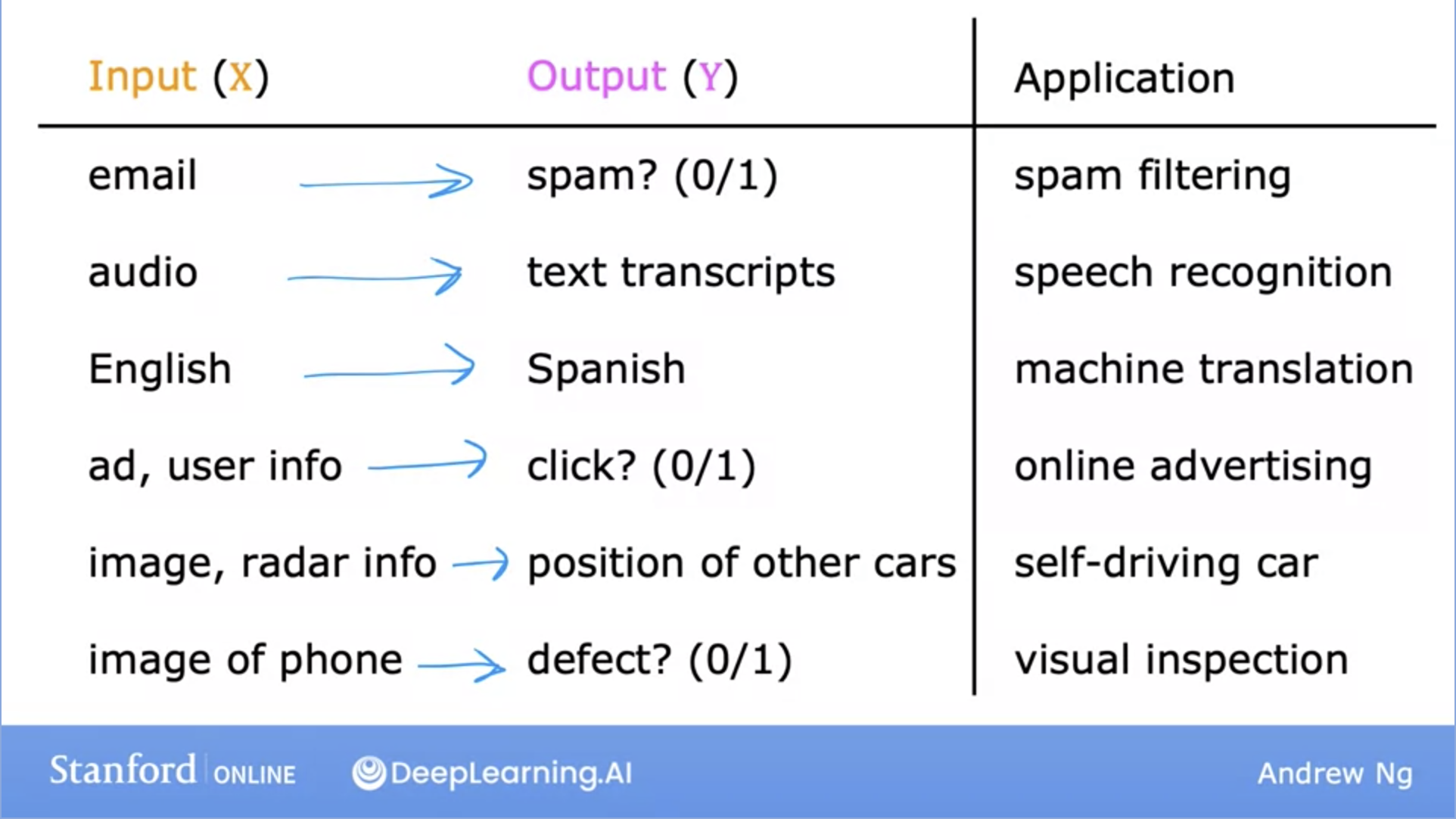• Let’s dive deeper into a specific example: Housing price prediction.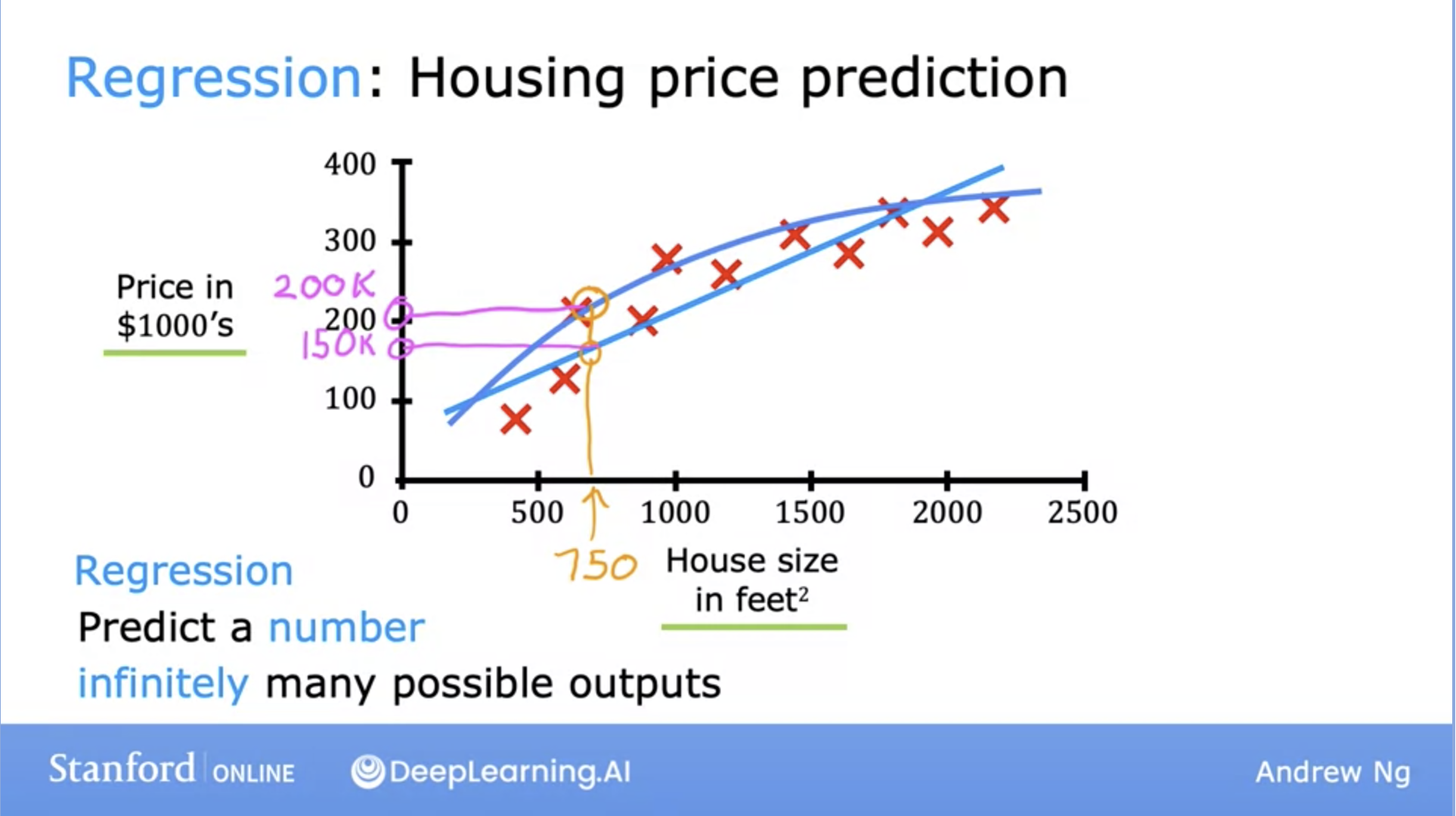• Here, the $$x$$ (input) value is the House size in feet squared and the $$y$$ (output) is the Price in \$1000’s.
• So how can the learning algorithm help us predict house values?
• One thing it can do is fit a straight, or in this case, a curved line that fits the data distribution well.
• Furthermore, this housing price prediction problem is a specific type of supervised learning known as regression.
• Regression: Predict a number from infinitely possible outputs.

### Linear Regression

• Fits a straight line through the dataset like the example below: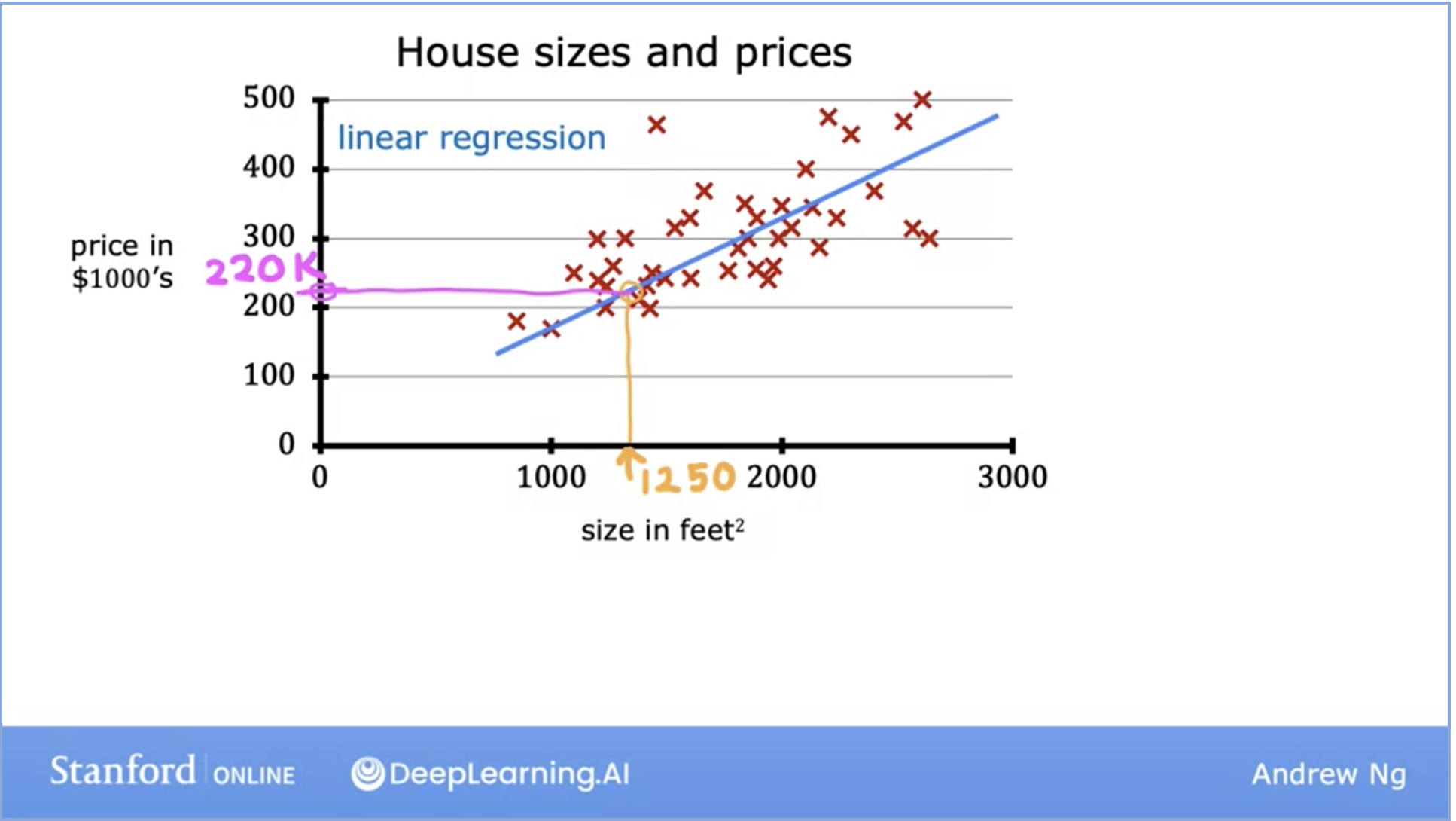• This is a regression model because it predicts numbers, specifically house prices per size.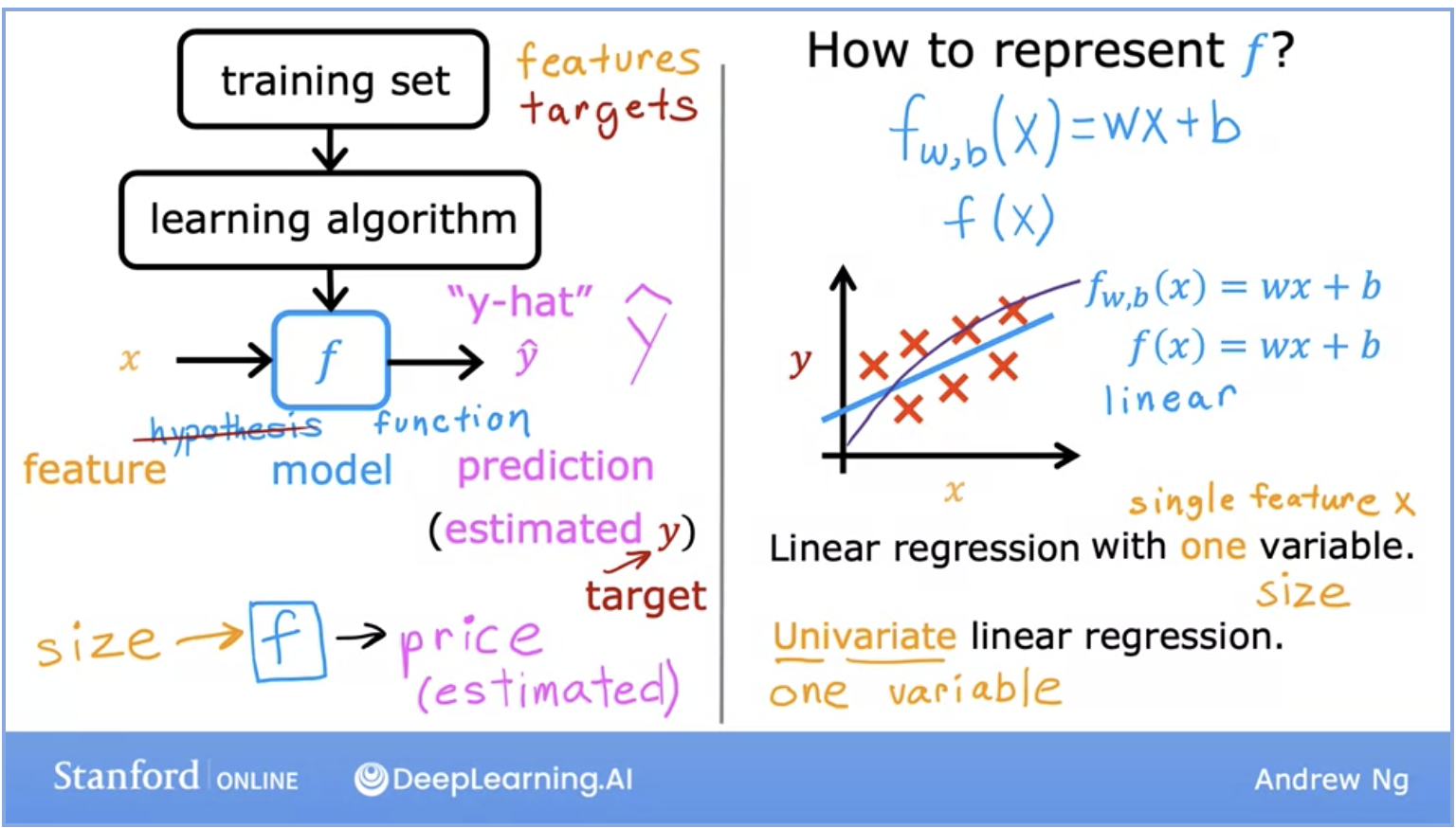• Above we can see the breakdown of the life cycle of how a model works.
• We start with a training set (with features and targets) that is fed into the learning algorithm.
• The learning algorithm then produces some function $$f$$, which is also called hypothesis in the Stanford ML lectures. This function, which is also the actual model, is fed the features and returns the prediction for the output $$y$$.
• What if our training set had multiple features as input parameters?
• Here we take the features $$w$$ as a row vector.
• $$b$$ is a single number.
• Multiple linear regression is the model for multiple input features. The formula is displayed below: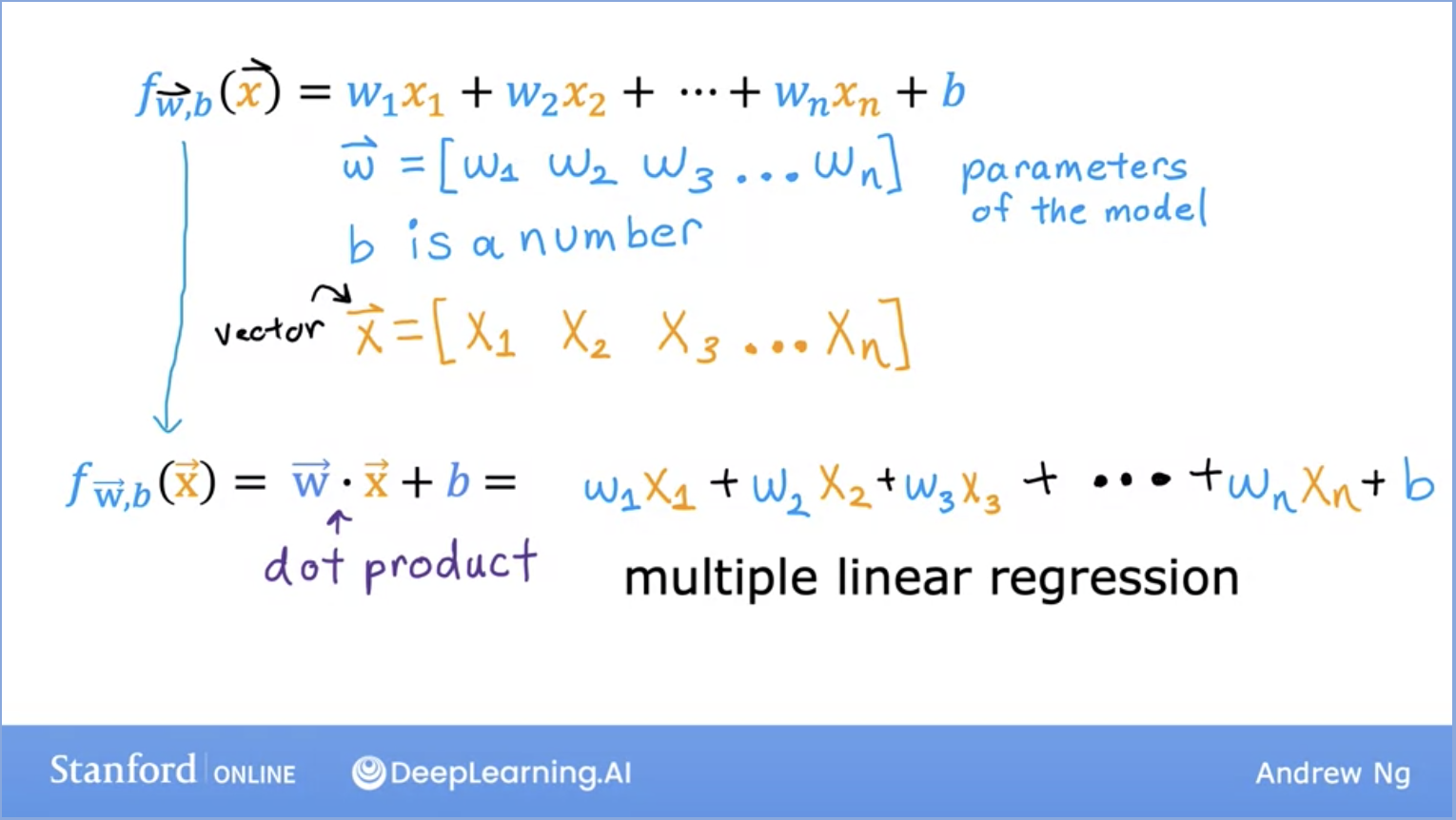#### Cost Function for Linear Regression (Squared error)

• In order to implement linear regression, we need to first define the cost function.
• The cost function tells us how well the model is doing, so we can improve it further.
• Just a bit of context and recap before we dive into what the cost function is:
• Model: is the $$f$$ function created by our learning algorithm represented as $$f(x) = wx + b$$ for linear regression.
• $$w,b$$ here are called parameters, or coefficients, or weights. These terms are used interchangeably.
• Depending on what the values of $$w,b$$ are, our function changes.
• Now lets jump back to the cost function! The cost function is essentially trying to find $$w,b$$ such that the predicted value of y (also known as \hat{y}) is as close to the actual value for y for all the values of $$(x,y)$$.
• What does the cost function do, mathematically speaking? Lets look at the image below: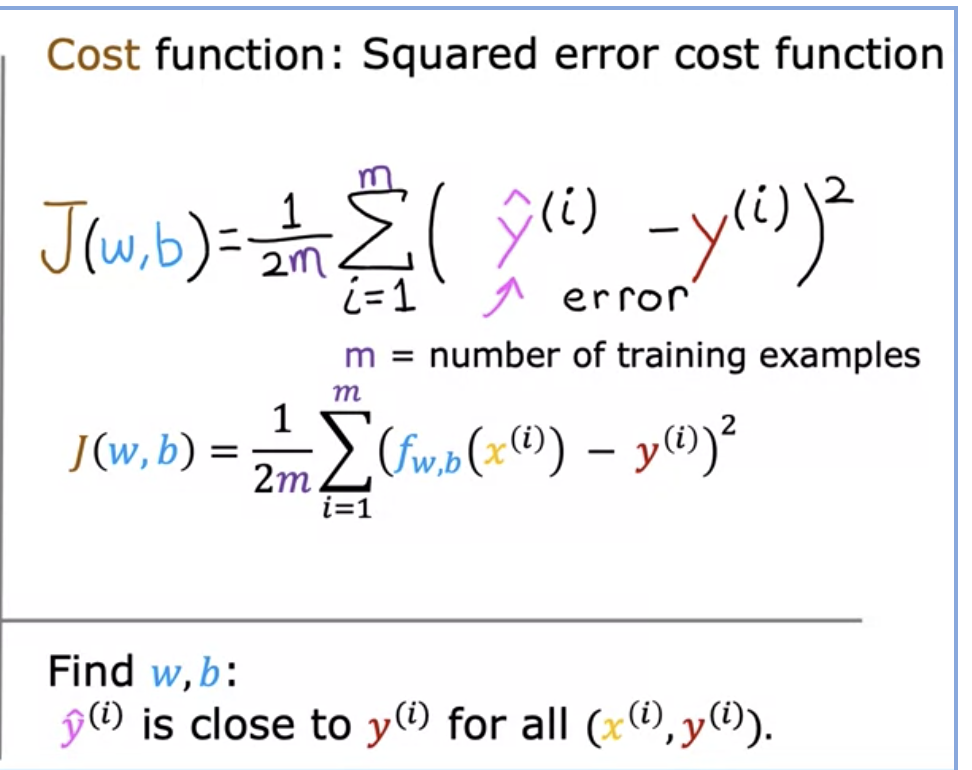• Lets break down what each of these terms mean here in the formula above.
• It takes the prediction $$\hat{y}$$ and compares it to the target $$y$$ by taking $$\hat{y} - y$$.
• This difference is called error, aka how far off our prediction is from the target.
• Next, we will compute the square of this error. We will do this because we will want to compute this value from different training examples in the training set.
• Finally, we want to measure the error across the entire training set. Thus, we will sum up the squared error.
• To build a cost function that doesn’t automatically get bigger as the training set size gets larger by convention, we will compute the average squared error instead of the total squared error, and we do that by dividing by $$m$$ like this.
• The last part remaining here is that by convention, the cost function that is used in ML divides by 2 times $$m$$. This extra division by 2 is to make sure our later calculations look neater, but the cost function is still effective if this step is disregarded.
• $$J(w,b)$$ is the cost function and is also called the squared error cost function since we are taking the squared error of these terms.
• The squared error cost function is by far the most commonly used cost function for linear regression, and for all regression problems at large.
• Let’s talk about what the cost function does and how it is used.
• The cost function measures how well a line fits the training data.
• Goal: Find the parameters $$w$$ or $$w,b$$ that result in the smallest possible value for the cost function $$J$$. Keep in mind the cost function $$J$$ will not be in a 1D space, so minimizing this is not an easy task.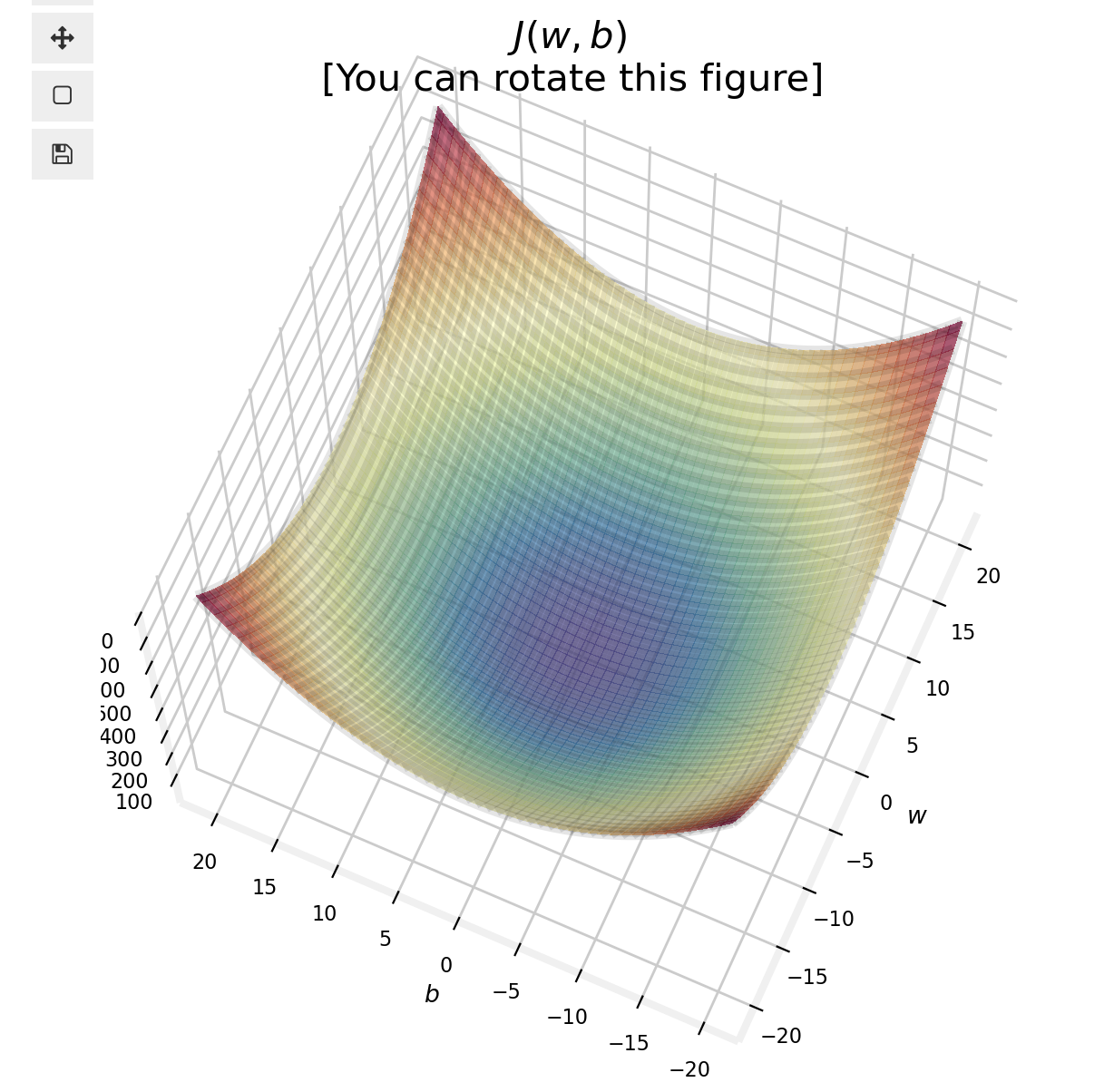• We will use batch gradient descent here; gradient descent and its variations are used to train, not just linear regression, but other more common models in AI.

#### Gradient Descent or Stochastic Gradient Descent

• Gradient descent can be used to minimize any function, but here we will use it to minimize our cost function for linear regression.
• Let’s outline on a high level, how this algorithm works:
• Start with some parameters $$w,b$$.
• Computes gradient using a single Training example.
• Keep changing the values for $$w,b$$ to reduce the cost function $$J(w,b)$$.
• Continue until we settle at or near a minimum. Note, some functions may have more than 1 minimum.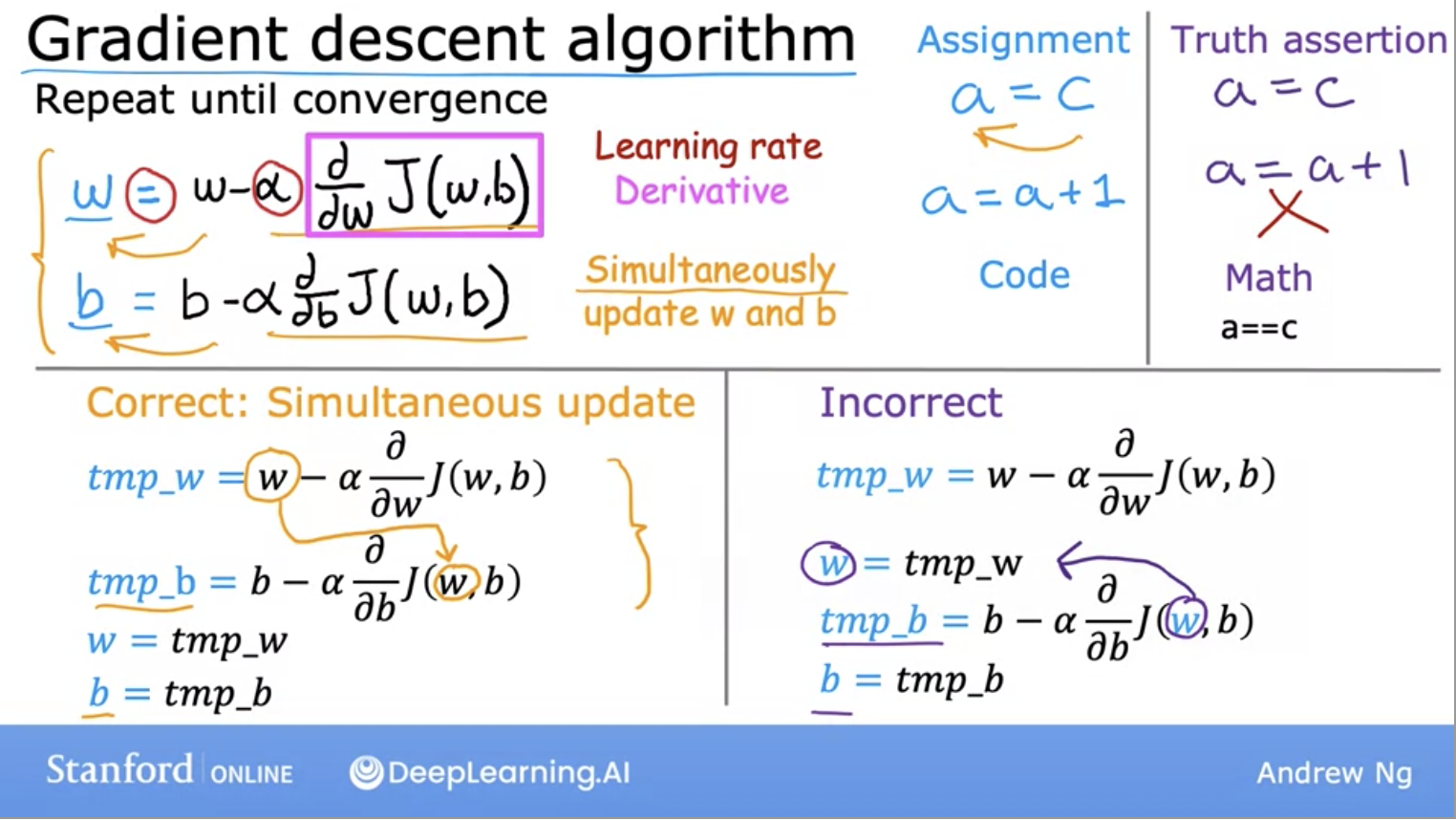• Above is the gradient descent algorithm. Note the learning rate alpha, it determines how big of a step you take when updating $$w$$ or $$b$$.
• If the learning rate is too small, you end up taking too many steps to hit the local minimum which is inefficient. Gradient descent will work but it will be too slow.
• If the learning rate is too large, you may take a step that is too big as miss the minimum. Gradient descent will fail to converge.
• How should we choose the learning rate then? A few good values to start off with are $$0.001, 0.01, 0.1, 1$$ and so on.
• For each value, you might just run gradient descent for a handful of iterations and plot the cost function $$J$$ as a function of the number of iterations.
• After picking a few values of the learning rate, you may pick the value that seems to decrease the learning rate rapidly.
• How do we know we are close to the local minimum? Via a game of hot and cold because as we get near a local minimum, the derivative becomes smaller. Update steps towards the local minimum become smaller, thus, we can reach the minimum without decreasing the learning rate.
• How can we check gradient descent is working correctly?
• We can have 2 ways to achieve this. We can plot the cost function $$J$$, which is calculated on the training set, and plot the value of $$J$$ at each iteration (aka each simultaneous update of parameters $$w,b$$) of gradient descent.
• We can also use an Automatic convergence test. We choose an $$\epsilon$$ to be a very small number. If the cost $$J$$ decreases by less than $$\epsilon$$ on one iteration, then you’re likely on this flattened part of the curve, and you can declare convergence.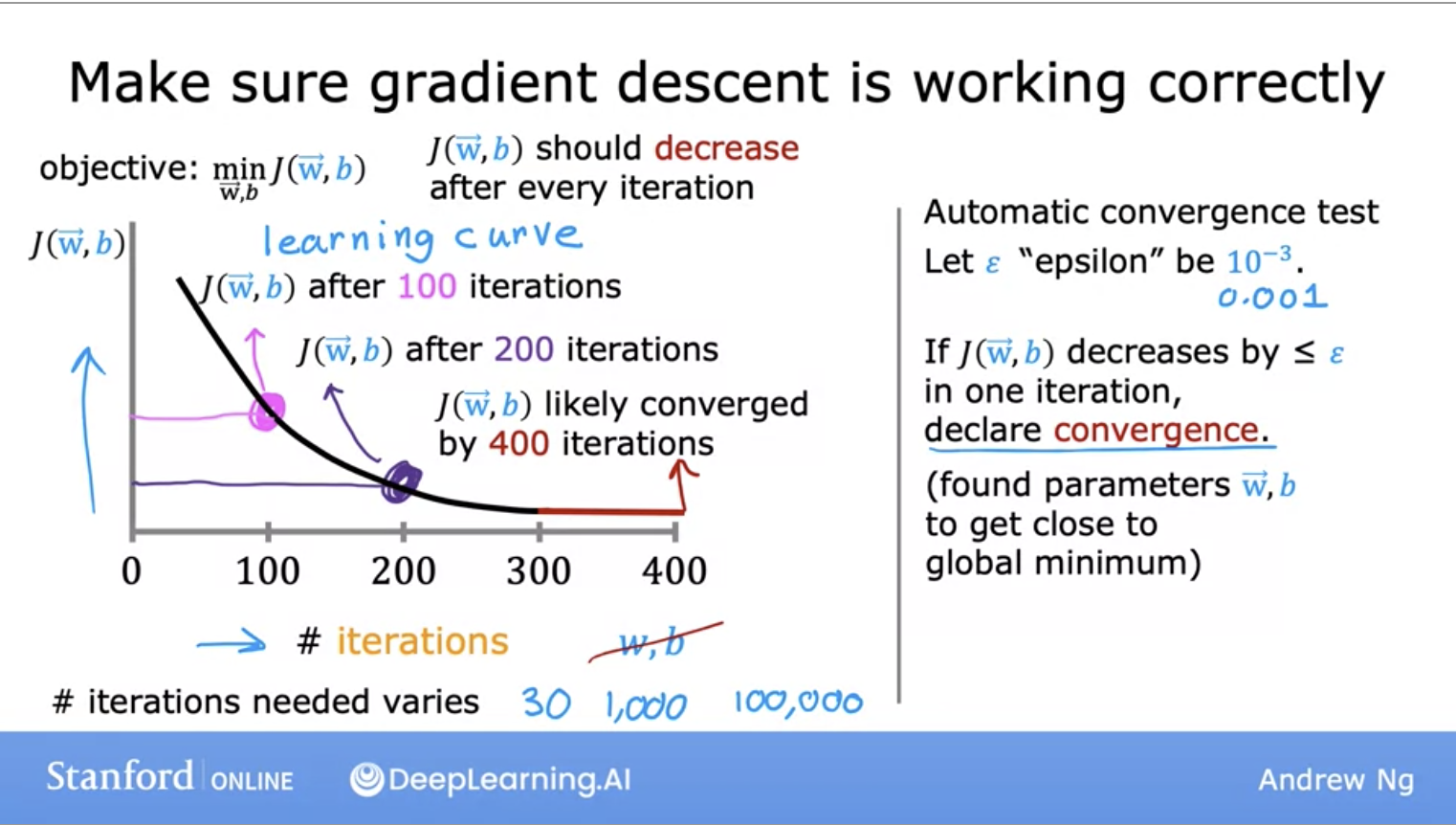• Adam algorithm can see if our learning rate is too small and we are just taking tiny little steps in a similar direction over and over again. It will make the learning rate larger in this case.
• On the other hand, if our learning rate is too big, where we are oscillating back and forth with each step we take, the Adam algorithm can automatically shrink the learning rate.
• Adam algorithm can adjust the learning rate automatically. It uses different, unique learning rates for all of your parameters instead of a single global learning rate.
• Below is the code for the Adam algorithm: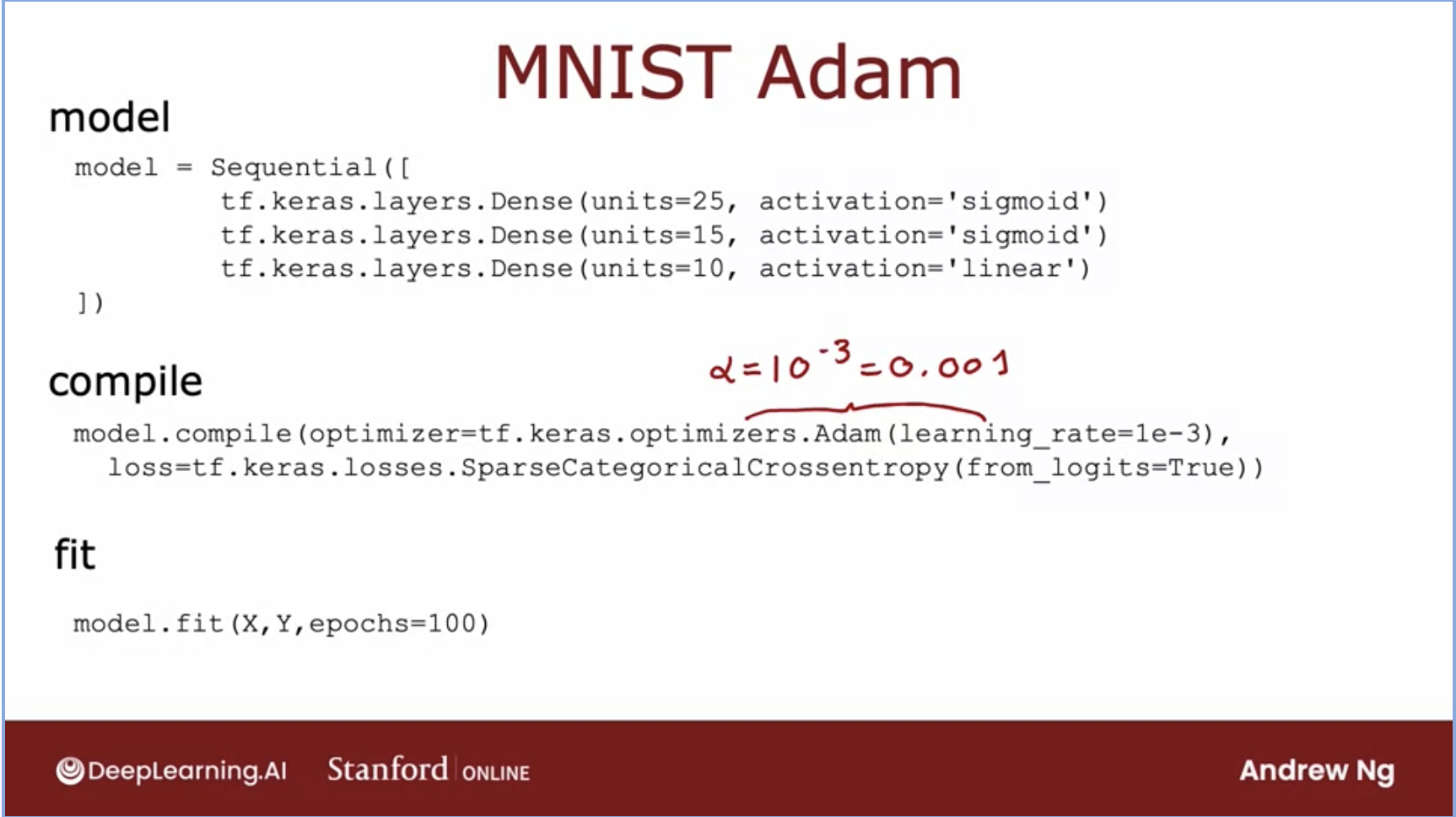#### Feature Scaling

• Lets look at some techniques that make gradient descent work much better, we will start with a technique called feature scaling.
• This will enable gradient decent to run much faster.
• What feature scaling does is that it makes the features involved in the gradient descent computation are all on the similar scale.
• This ensures that the gradient descent moves smoothly towards the minima and that the steps for gradient descent are updated at the same rate for all the features.
• Having features on a similar scale helps gradient descent converge more quickly towards the minima.

#### Batch Gradient Descent

• Every step of gradient descent, we look at all the training examples instead of a subset of them.
• Batch gradient descent is an expensive operation since it involves calculations over the full training set at each step. However, if the function is convex or relatively smooth, this is the best option.
• Batch G.D. also scales very well with a large number of features.
• Computes gradient using the entire Training sample.
• Gives optimal solution if its given sufficient time to converge and it will not escape the shallow local minima easily, whereas S.G.D. can.

### Polynomial Regression

• We’ve seen the idea of fitting a straight line to our data with linear regression, now lets take a look at polynomial regression which will allow you to fit curves and non linear functions to your data.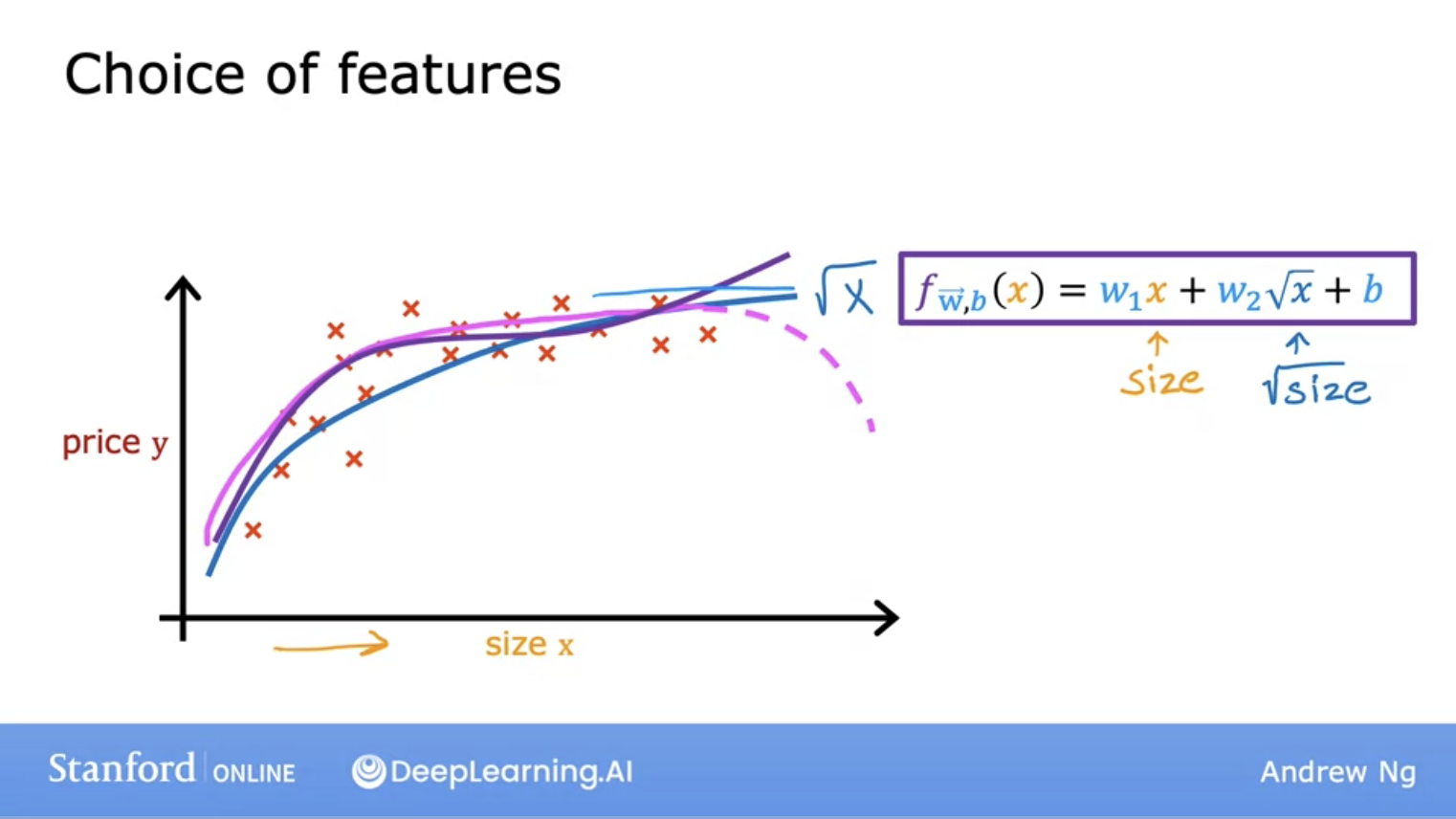## All 3 in one! (Cost function for Linear regression via Gradient descent)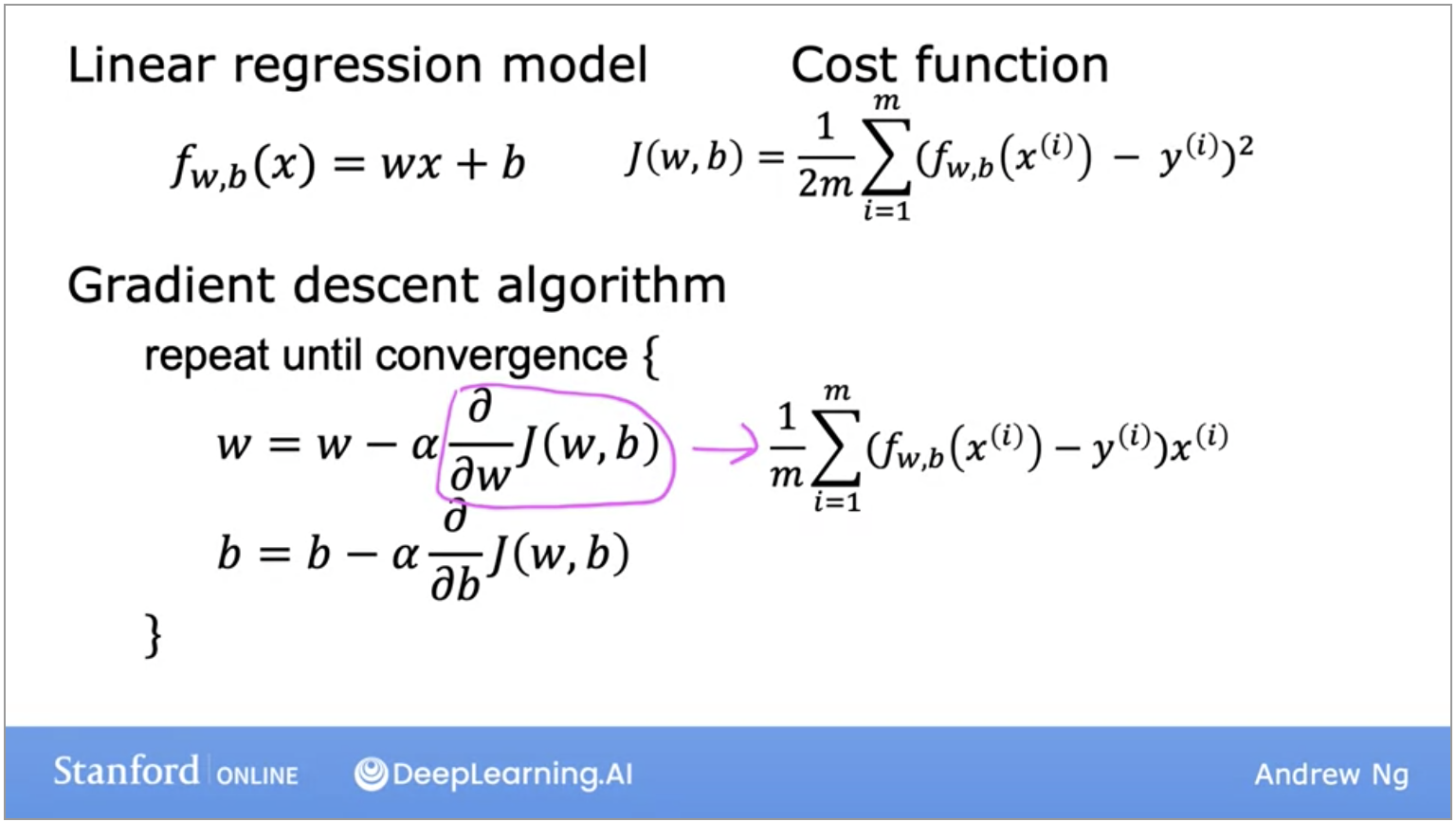• Quick recap of the formulas we’ve seen thus far in the image above.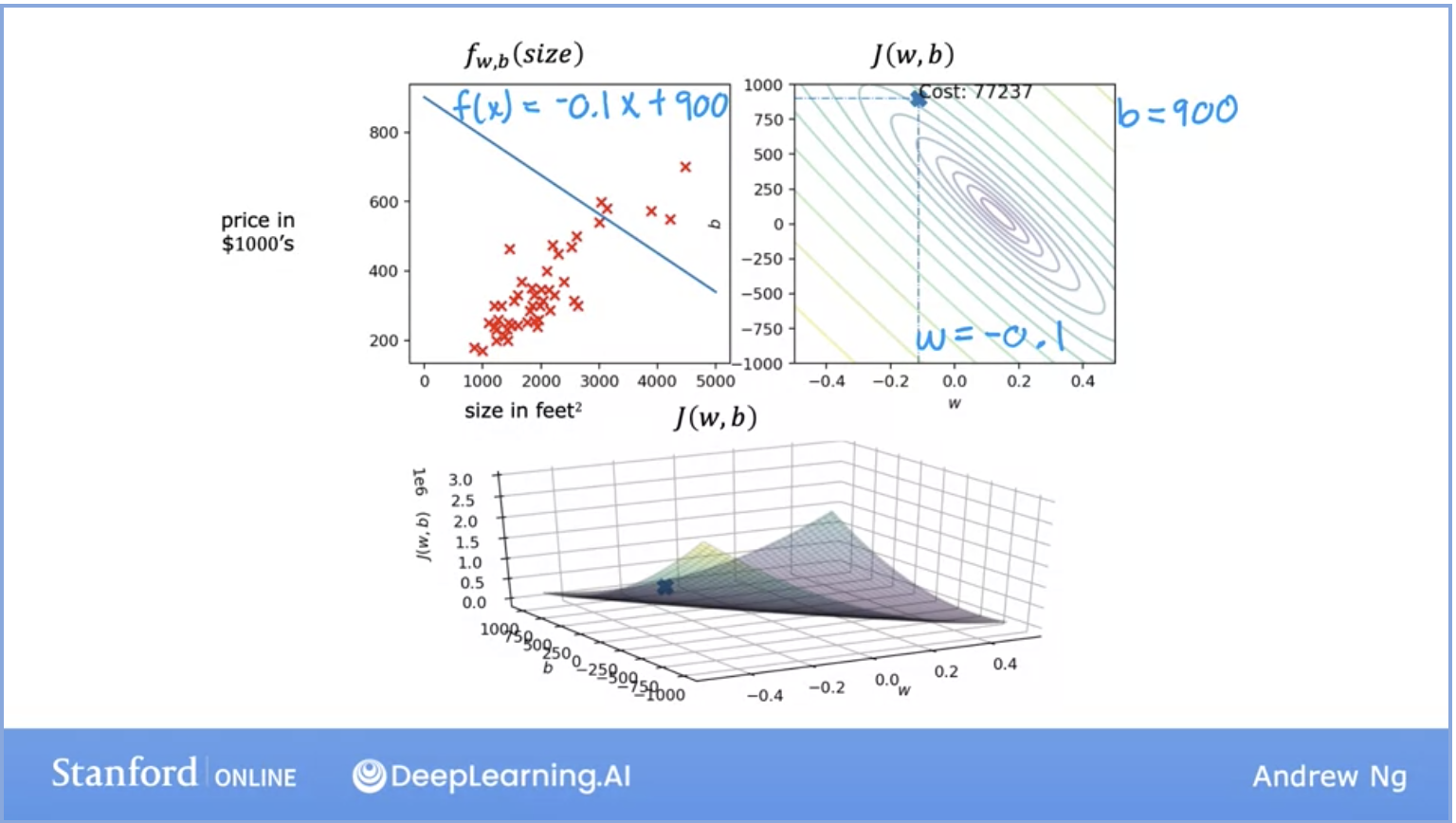- In the above image, on the left, we see the model representation with all of its data set. On the right and the bottom we see convex and surface representations of the cost function.

## Supervised Learning: Classification

• Another type of supervised learning algorithm is called the classification algorithm. Classification model predicts categories where there are only a small number of possible outputs.
• How is this different from regression we saw earlier? Let’s take a look with an example: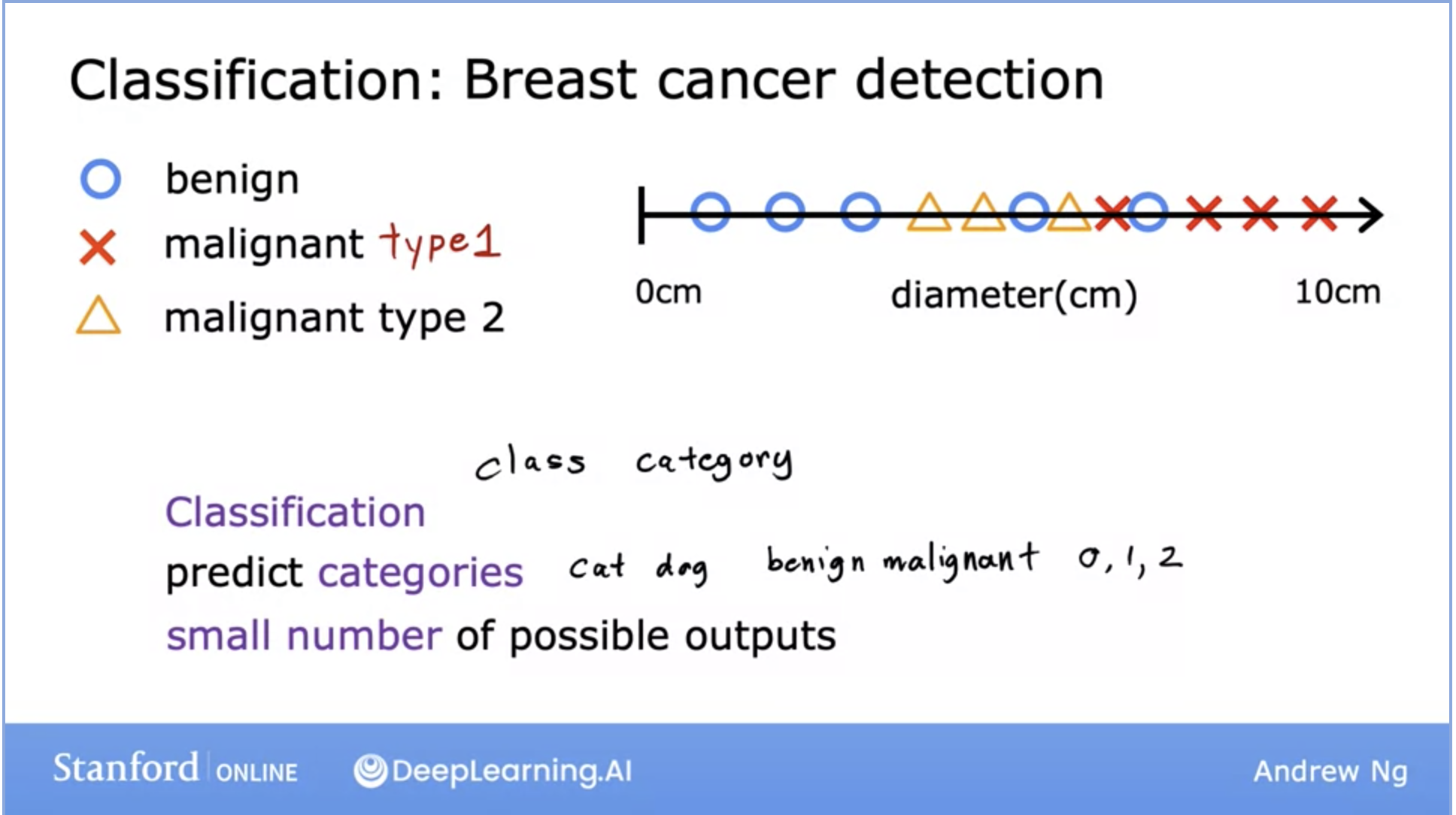• Above, we can see the breast cancer detection problem. Early detection can help lead to a cure in many cases and thus, this is a problem that’s currently being worked on.
• Why is this a classification problem? Because the output here is a definitive prediction of a class (aka category), either the cancer is malignant or it is benign.
• This is a binary classification, however, your model can return more categories like the image above. It can return different/specific types of malignant tumors.
• Classification predicts a small number of possible outputs or categories, but not all possible categories in between like regression does.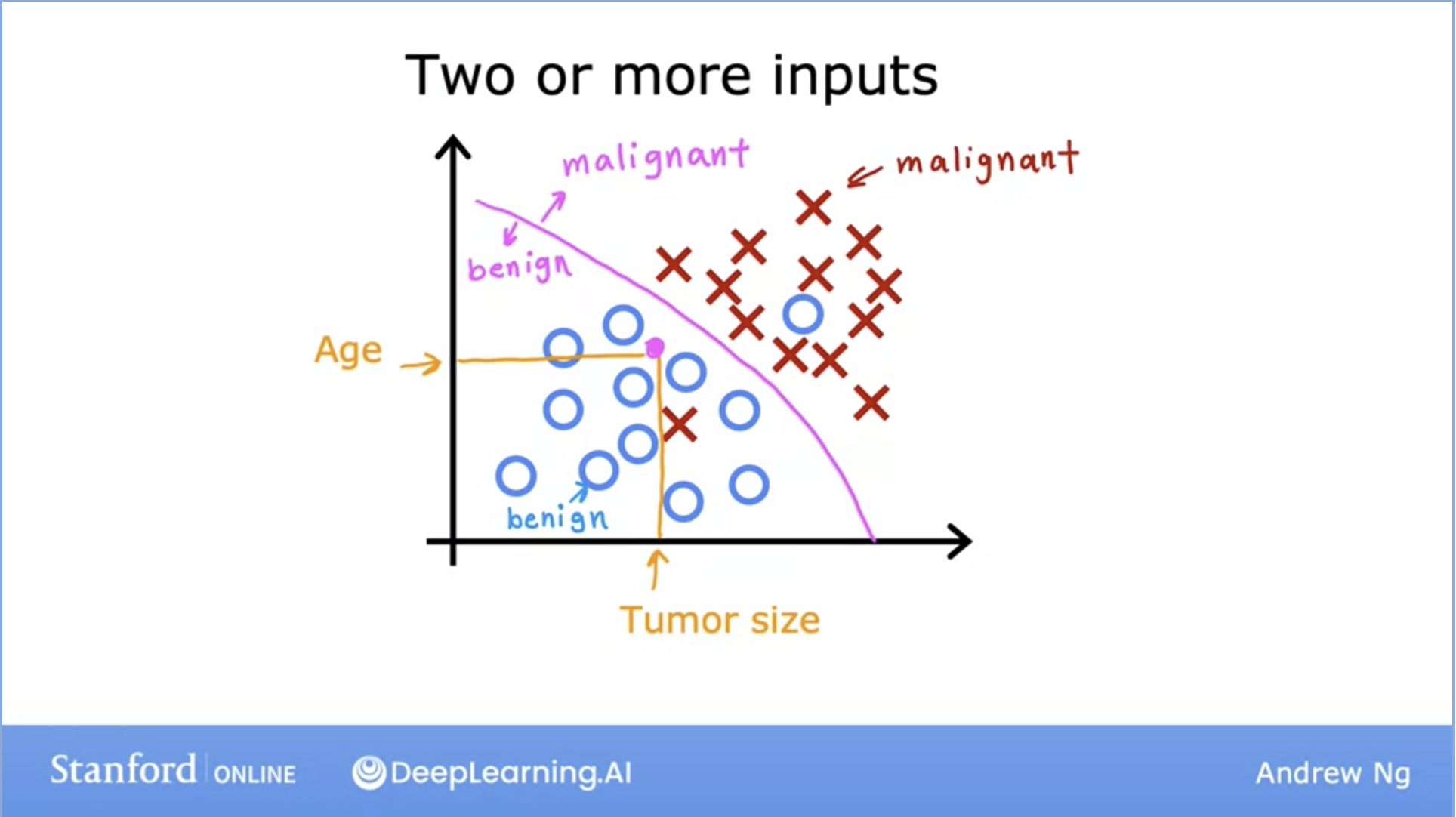• So far we’ve only seen datasets with one input, in reality, you can have two or more inputs given to the model as displayed above.
• In this scenario, our learning algorithm might try to find some boundary between the malignant tumor vs the benign one in order to make an accurate prediction.
• The learning algorithm has to decide how to fit the boundary line.
• Linear regression algorithm does not work well for classification problems. We can look at the image below, in the case of an outlier x, linear regression will have a worse fit to the data set and move its decision boundary to the right. The blue vertical decision boundary is supposed to be the decision between malignant and non-malignant.
• However, once we add the outlier data, the decision boundary changes and thus, the classification between malignant and non-malignant changes, thus giving us a much worse learned function.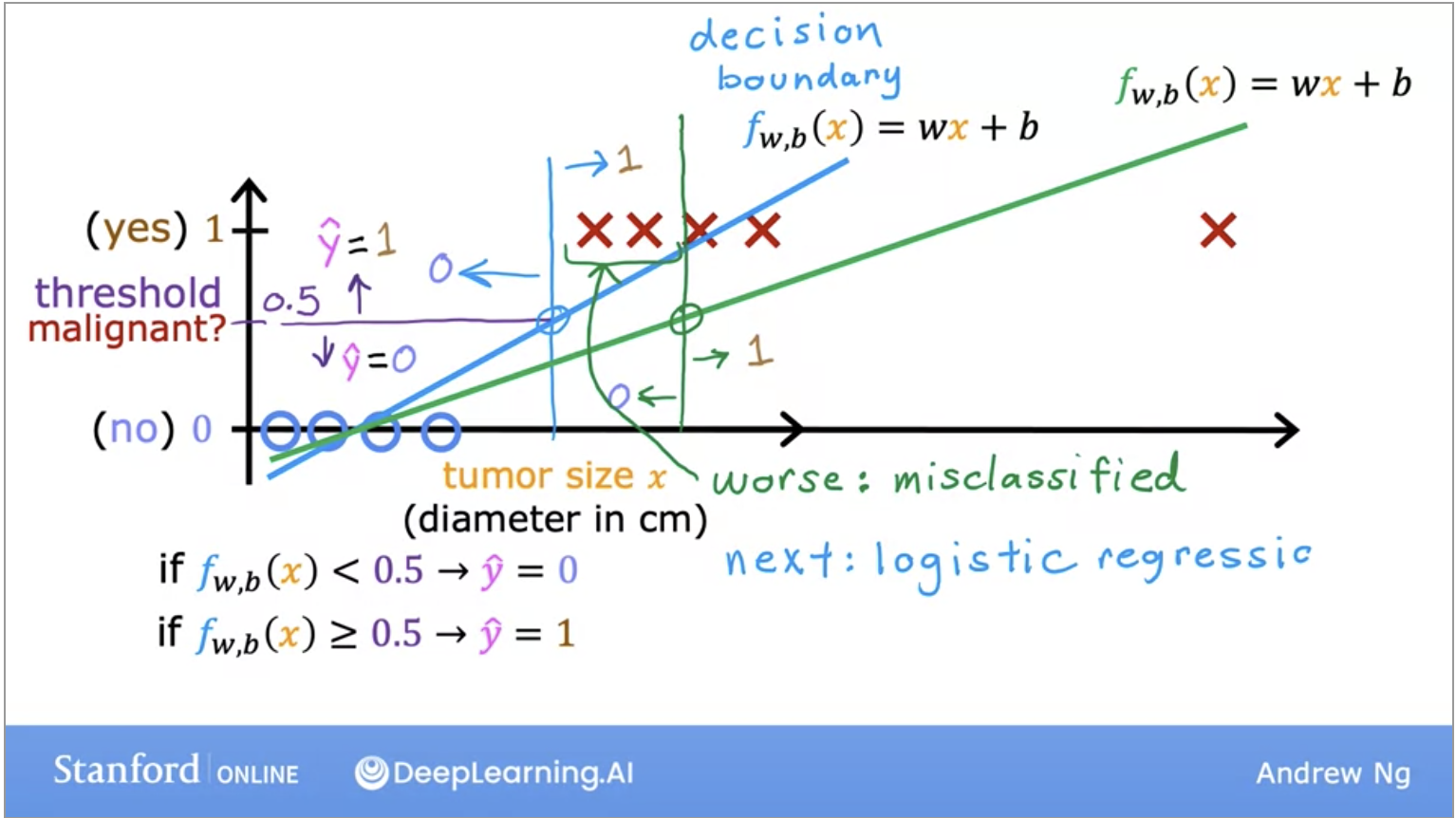### Logistic Regression

• The solution to our classification problem! Don’t get confused by its name, regression here is a misnomer, it’s used for classification problems because it returns a value between 0 or 1.
• To build out the logistic regression algorithm, we use the Sigmoid function because it is able to return us an output between 0 and 1.
• Lets look at its representation in the image below: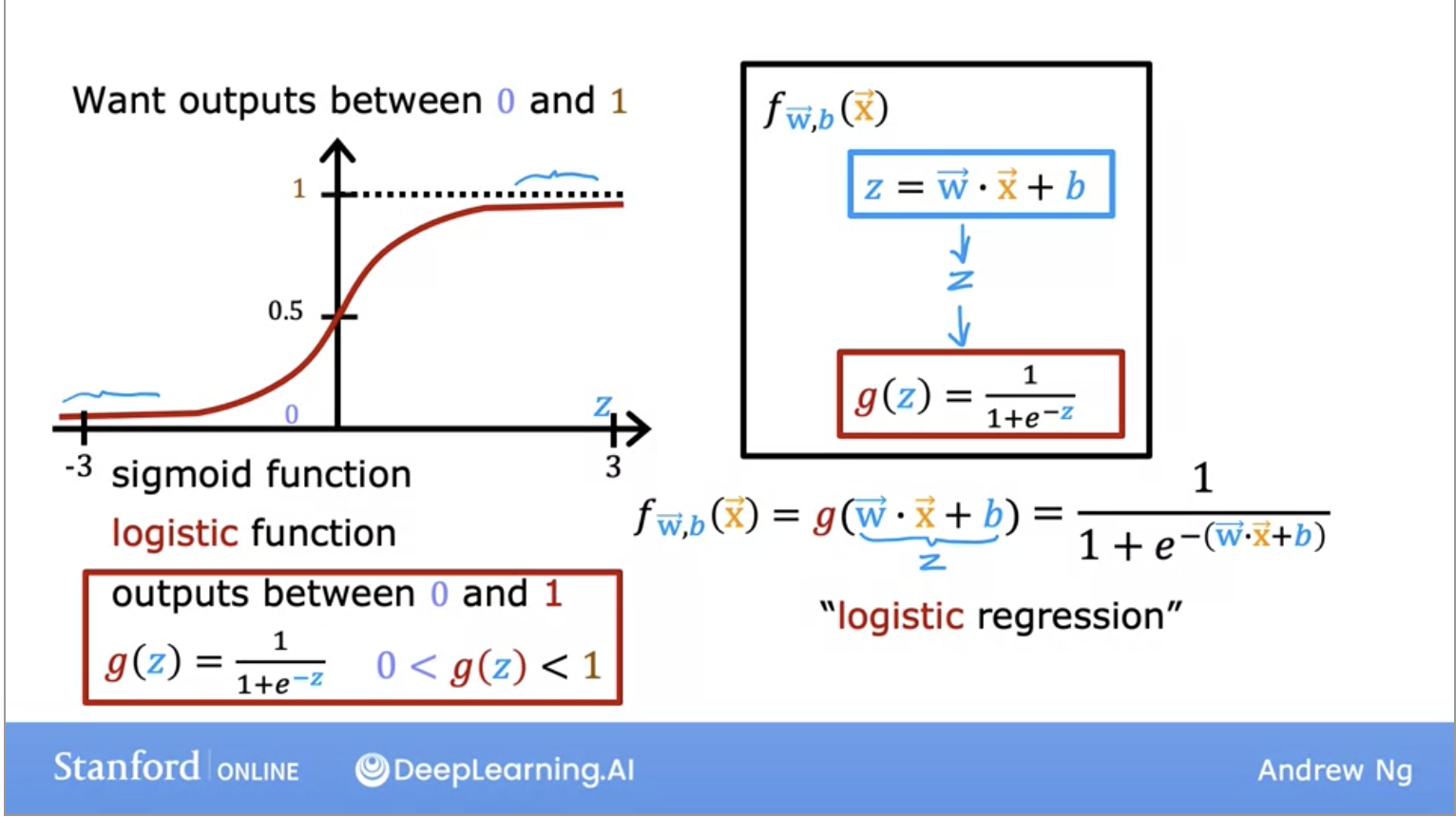• We can think of the output as a “probability” which tells us which class the output maps to.
• So if our output is between 0 and 1, how do we know which class (malignant or not-malignant) the output maps to?
• The common answer is to pick a threshold of 0.5, which will serve as our decision boundary.
• We can also have non-linear decision boundaries like seen in the image below: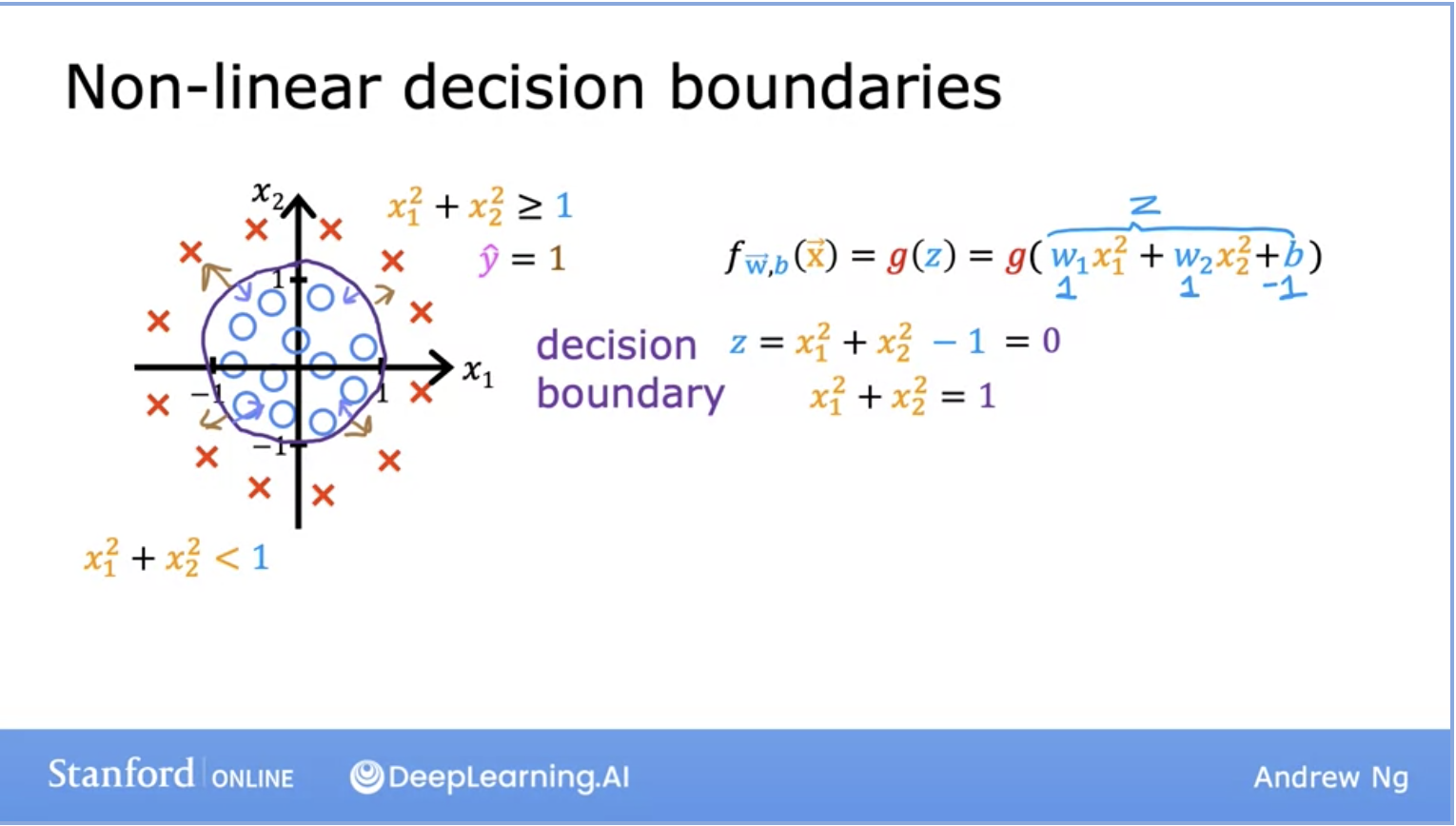#### Gradient Descent for Logistic Regression

• Remember this is the algorithm to minimize the cost function.
• One thing to note here is that even though the formula for gradient descent might look the same as it did when we were looking at linear regression, the function $$f$$ has changed: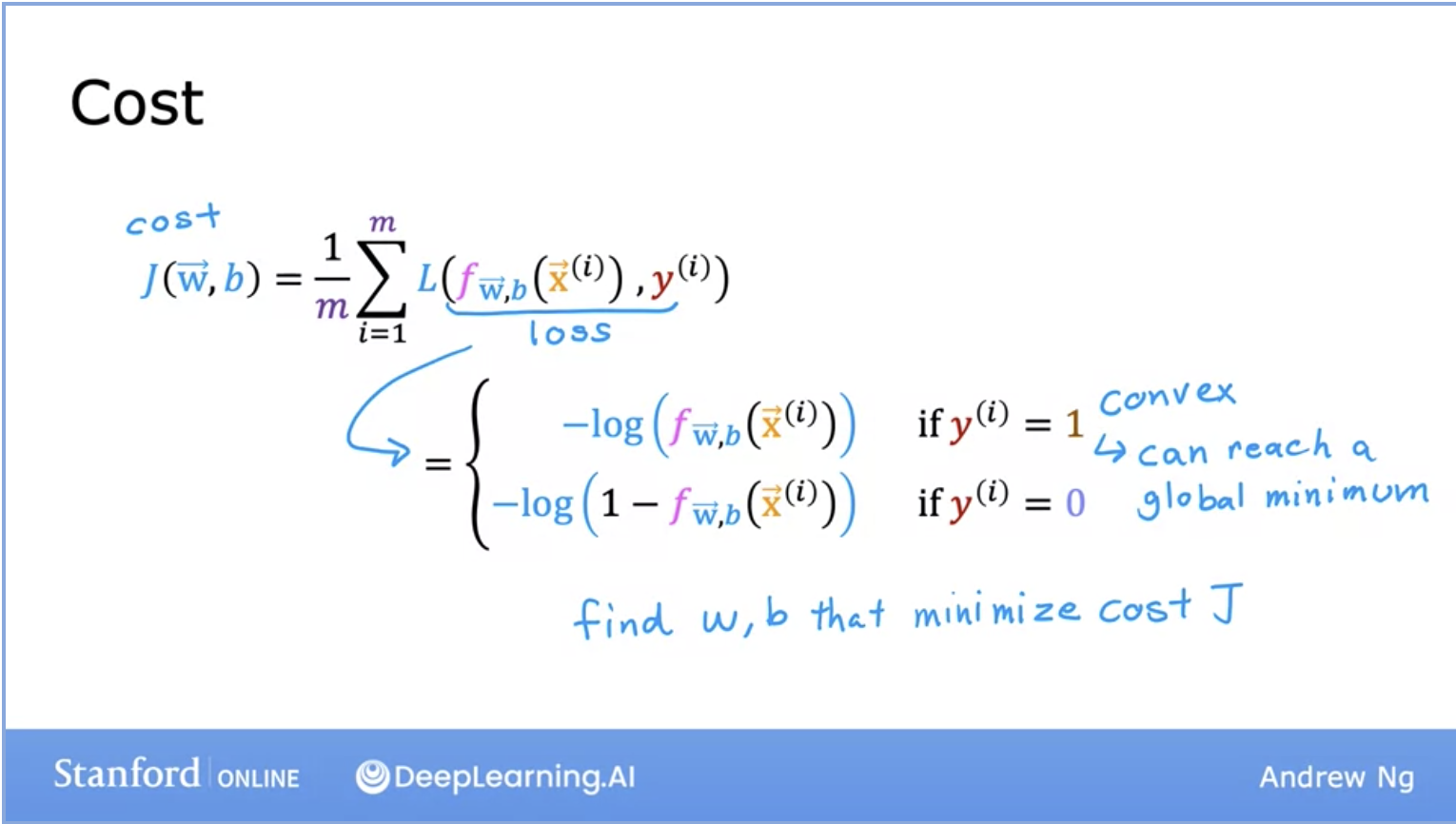#### Cost Function for Logistic Regression (Log Loss)

• One thing to keep in mind is Loss and Cost function mean the same thing and are used interchangeably.
• Below lets look at the function for the Logistic loss function. Remember that the loss function gives you a way to measure how well a specific set of parameters fits the training data. Thereby gives you a way to try to choose better parameters.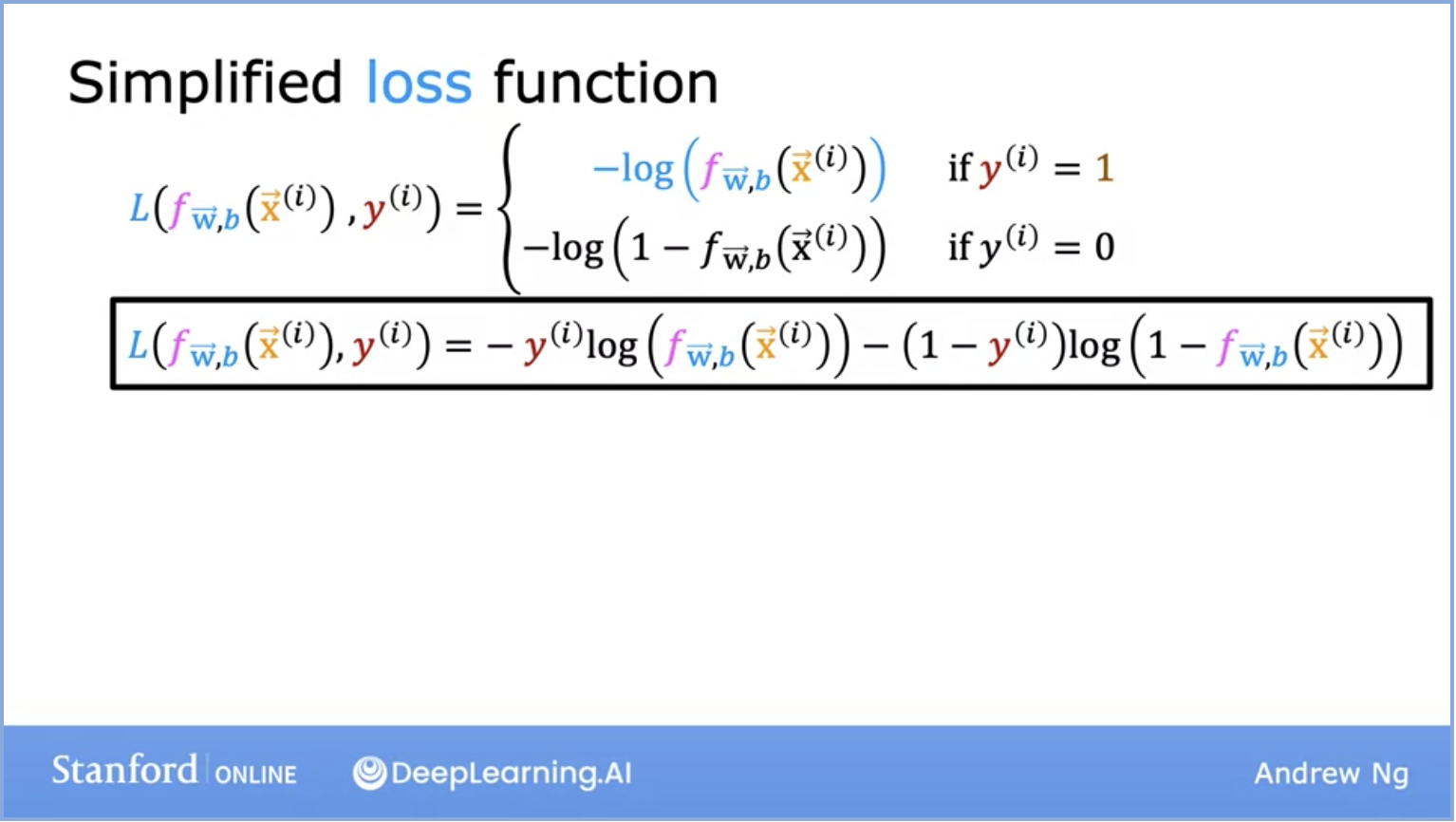• Note y is either 0 or 1 because we are looking at a binary classification in the image below. This is a simplified loss function.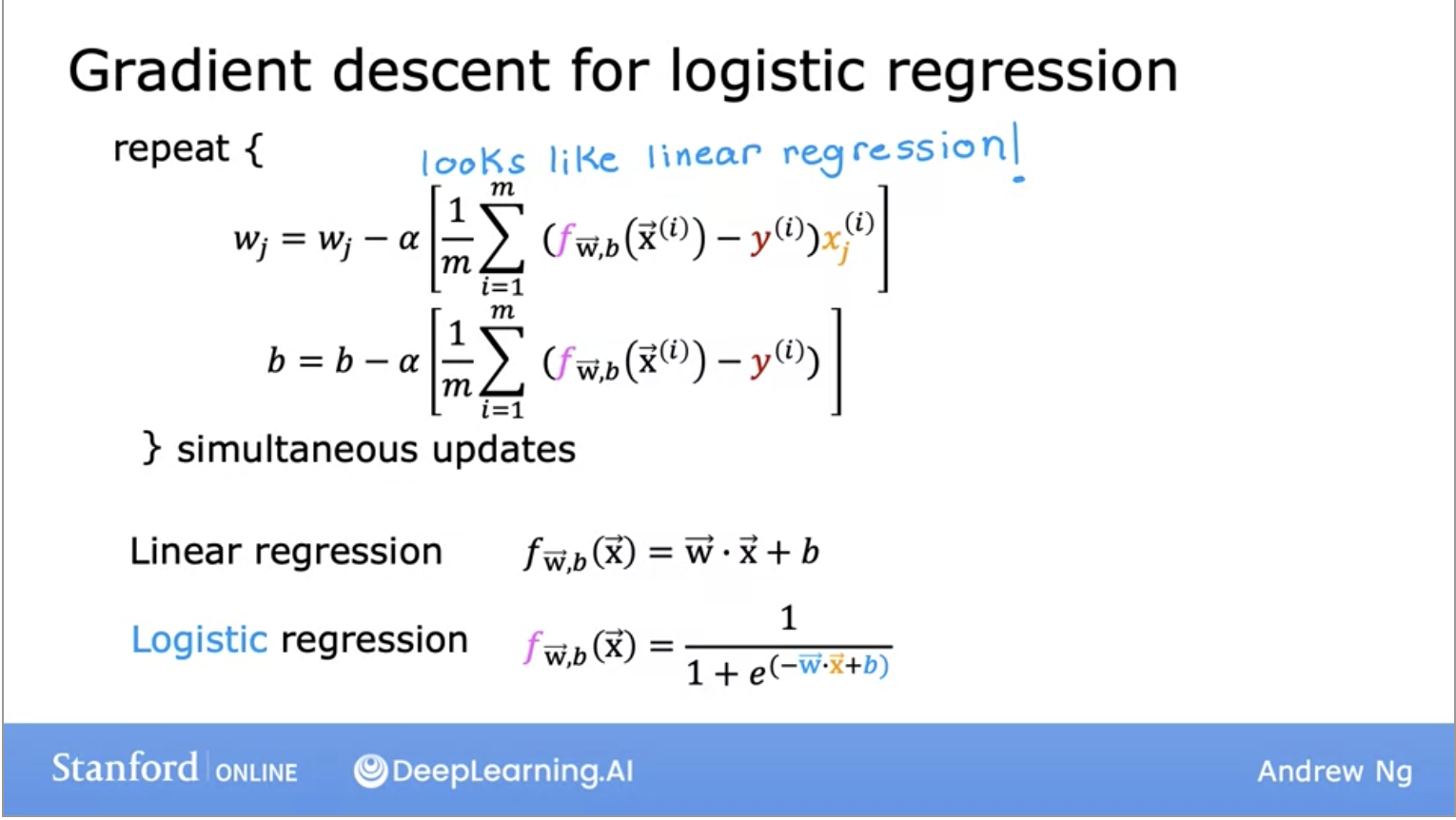• Even though the formula’s are different, the baseline of gradient descent are the same. We will be monitoring gradient descent with the learning curve and implementing with vectorization.

## Overfitting and Underfitting

• Overfitting is a problem we see when our data “memorizes” the training data. It will perform very well for training data but when it will not generalize well (not have the same accuracy for the validation set). The cost function might actually be = 0 in this case. The model here has high variance.
• Viable solutions for Overfitting:
• Collect more training examples. With a larger training set, the learning function will be able to fit the dataset better.
• Select features. See if you can use fewer or more features depending on your current model. If you have a lot of features and insufficient data, you may result in overfitting. Instead, you can select a subset of those features to use given the disadvantage of loosing some useful features.
• Regularization: reduce the size of parameters. Gently reduces the impact of certain features. Lets you keep all your features but doesn’t allow any one feature to have a large impact.
• Keep in mind, you don’t need to regularize $$b$$, usually just regularize $$w$$
• Underfitting is when we have high bias and the data does not fit the training data set well.
• Viable solutions for underfitting:
• Get more training data.
• Increase the size or number of parameters in the model.
• Increase the complexity of the model.
• Increasing the training time, until cost function is minimized.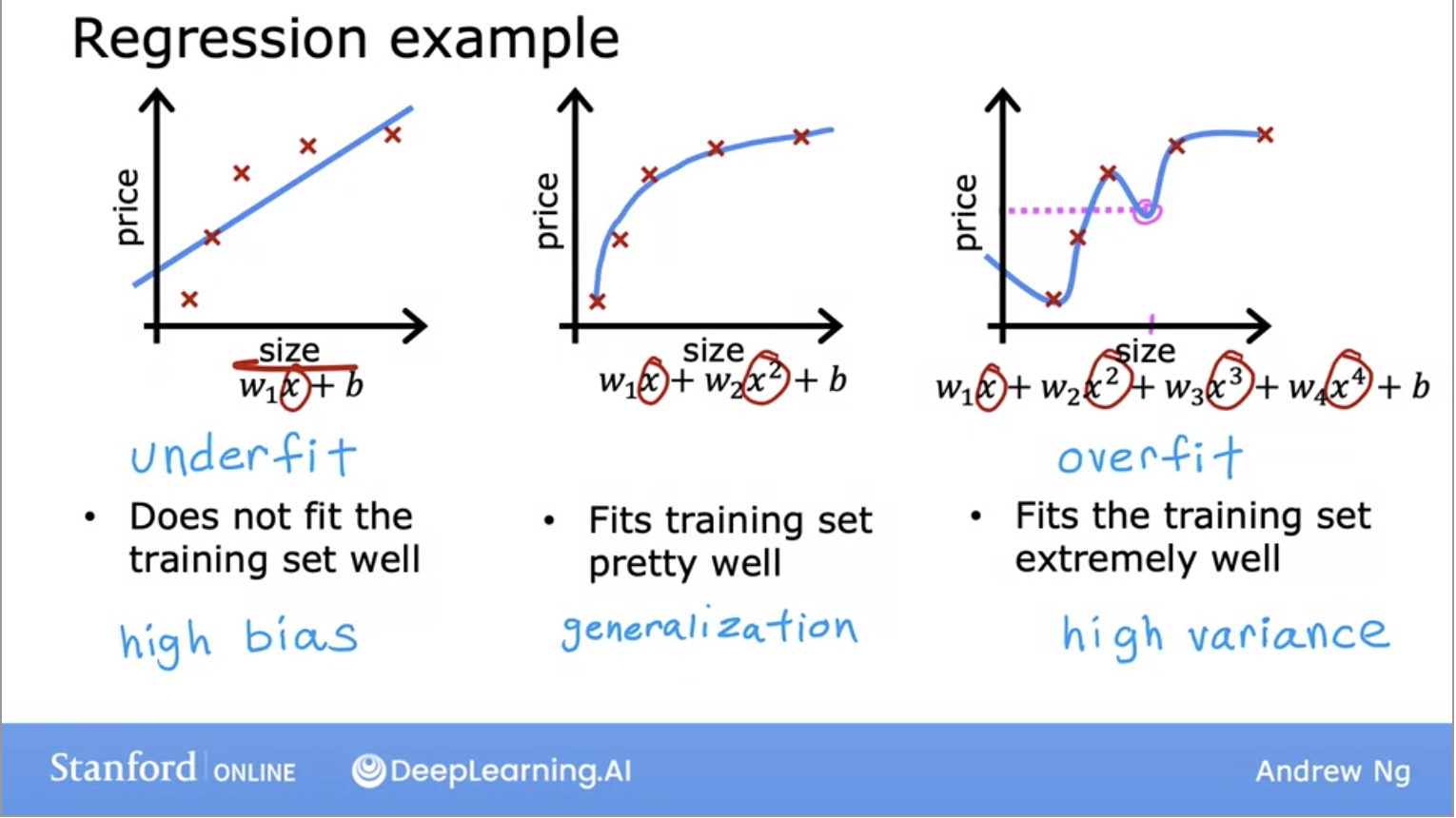• The middle model above is the goldilocks “just right” model as it generalizes well.

## TL;DR

• Lets quickly go over the key takeaways from this section:
• Supervised learning maps $$x$$ the input to the output $$y$$ and learns from being given the “right answers”. This is also called training your model.
• The two major types of supervised learning algorithms are regression and classification.
• Regression: Predict a number from infinitely many possible outputs.
• Linear Regression is a type of regression where the model’s cost function is fit with a linear line.
• The model is represented by a function, with parameters (w,b), and the cost function’s goal is to minimize $$J(w,b)$$ to find the best fitting line through the data set.
• Classification: Predict a category for a finite number of possible outputs.